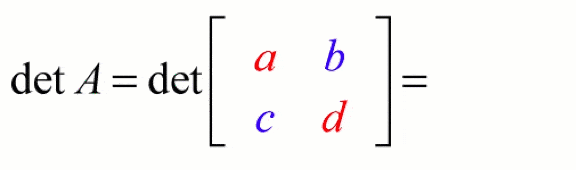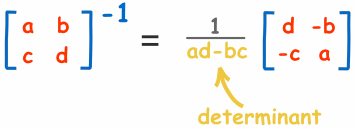Covers: theory of Matrix Algebra
Estimated time needed to finish: 23 minutes
• What is matrix determinant?
• What is matrix Eigendecomposition and what it is similar to (hint: PCA and SVD)?
• What are some special properties of positive-definite matrices?
• Do I need to know and understand all these operations to be a DL practitioner?
##### How to use this item?

In this video, you will learn about:

• Matrix Determinant
• Inversion
• Trace
• Eigendecomposition
• Positive Definite matrices
• Orthogonal & Symmetric matrices

Even though, therse linear argebra operations are good to be known, they are not frequentelly applied operations in DL. Anyway, It's very easy to implement them in PyTorch. However,or building simple networks like a fully connected networks with embedings, for categorical features, and numerical features, you won't need these operations.

Skimm through the comprehensive deffitions of the above terms here or one-sentace explanation bellow. Learn more by watching the video.

• Matrix Determinant: "The determinant of a square matrix is a single number that, among other things, can be related to the area or volume of a region. In particular, the determinant of a matrix reflects how the linear transformation associated with the matrix can scale or reflect objects." mathinsight.orgGIF Source: https://www.chilimath.com/

• Inversion This work is interesting, as it talks about importance of matrix inversion in veruy specific DL application• Trace Is the sum of all the diagonal elements present in a given matrix.• Eigendecomposition Is important in ML task for dimensionality reductions (PCA, or SVD, etc.). one of the application may be a recoonstruction, i.e. estimation of a full, sparse matrix. You can read more about Eigendecomposition in a post by machinelearningmastery.com• Positive Definite matrices Lead to convex functions. This property is useful in optiomization problems. Look at this paper, to see the appication in computer vision!

• Orthogonal & Symmetric matrices Useful properties in matrox decomposition, e.g. Eigendecomposition.

Fail to play? Open the link directly: https://youtu.be/K5jhYlGX4RY
Amir Hajian
0 comment
Recipe
publicShare
Star(0)

### Foundations Of Algebra For Deep Learning

ContributorsTotal time needed: ~2 hours
Objectives
You will learn fundamental PyTorch operations with tensors for future DL/NN applications.
Potential Use Cases
Building NN from scratch
Who is This For ?
INTERMEDIATE
Click on each of the following annotated items to see details.
Resources4/6
VIDEO 1. Tensors, Matrices, Dot Product
• What are the most basic matrix manipulation techniques I need to know?
• How easy does PyTorch make it to perform these operations?
19 minutes
VIDEO 2. Matrices and Eigen-decomposition
• What is matrix determinant?
• What is matrix Eigendecomposition and what it is similar to (hint: PCA and SVD)?
• What are some special properties of positive-definite matrices?
• Do I need to know and understand all these operations to be a DL practitioner?
23 minutes
VIDEO 3. Mathematical Non-linearities
• How to solve eigendecomposition on a whiteboard?
• What is the relevance of nonlinearities for deep learning?
23 minutes
REPO 4. Hands-on Linear Algebra for Deep Learning
• How to carry out linear algebraic tasks for deep learning in PyTorch?
30 minutes
RECIPE 5. Matrix Algebra
10 minutes
BOOK_CHAPTER 6. Linear Algebra for Deep Learning
• How is linear algebra used in deep learning?
20 minutes

0 comment